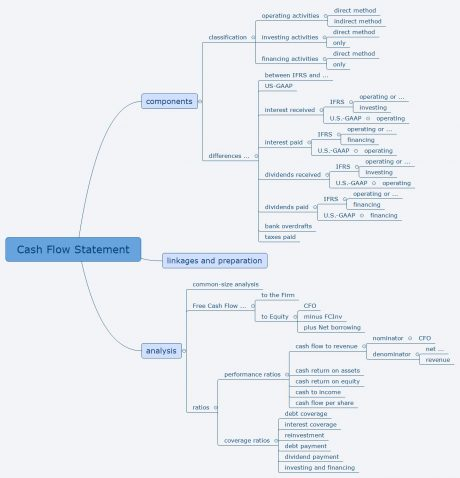Kontakt : |

CFA Level I, Financial Reporting and Analysis, Cash Flow Statement

There are two different ways to calculate the statement of cash flows, the direct format and

the indirect format.

Using the direct format, we calculate the cash flow as:

cash flow = incoming cash flows – outgoing cash flows.

However, when using the indirect format, we calculate the cash flow this way:

cash flow = net income

+ expenses that were not cash outflows

– revenues, that were not cash inflows

+ cash inflows, that were not revenues

– cash outflows that were not expenses

Let us take a look at an example to better understand the two concepts leading to the same result.

Example:

Company XY had sales \$8,000, but only 30% of them were paid in the same period. The salaries paid amounted to \$2,000, the interest paid was \$1,000. Loan was granted by banks at \$ 3,000, the principal payment on a different loan was \$800. Depreciation and amortization was \$700, the purchase of equipment amounted to \$1,200.

Calculate the cash flow, using the direct format as well as the indirect format.

Solution:

First, we need to compute net income. This is this done by

net income =  revenue – expenses

= 8,000 – (2,000 + 1,000 + 700)

= 8,000 – 3,700

= \$4,300.

Then, using the indirect format, we shall compute as follows:

net income 4,300

plus expenses that were not cash outflows +700

minus revenues, that were not cash inflows -5,600

plus cash inflows, that were not revenues +3,000

minus cash outflows that were not expenses -2,000

= cash flow. = +400.

Using the direct format, we compute the cash flow as follows:

cash flow =  cash inflow –  cash outflow

= 0.3*8,000 + 3,000 – (2,000 + 1,000 + 800 + 1,200)

= 5,400 – 5,000

= +400.

As promised, the two formats lead to the same result.

The most important points of Financial Reporting and Analysis, and Long-Lived Assets in this post can be summarised in this MindMap:Did you find the MindMap helpful? All Lambert Education MindMaps for CFA Level 1 and Level 2 can be purchased in the online shop at https://daniel-lambert.de/produkt-kategorie/chartered-financial-analyst/

0 responses on "CFA Level I, Financial Reporting and Analysis, Cash Flow Statement"

Über Uns

Wir bieten seit 1994 Unterricht für BWL, VWL, Statistik und Mathematik. Mit uns bereiten Sie sich optimal auf Ihre Prüfung vor - kurz, knapp und auf den Punkt gebracht. Sie haben bei uns die Möglichkeit der Gruppenkurse und des Einzelunterrichts, der Präsenzkurse und der Skriptsammlungen.

Wer ist Online?

Im Moment ist niemand online.